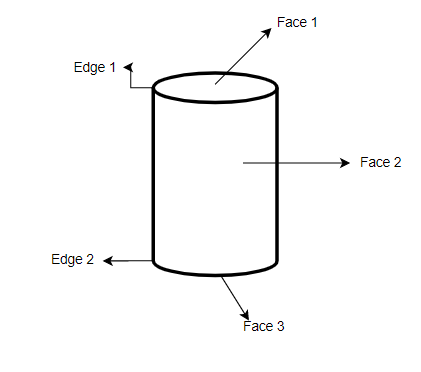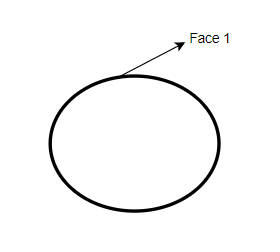Courses
Courses for Kids
Free study material
Offline Centres
MoreLast updated date: 09th Dec 2023
Total views: 333.6k
Views today: 8.33k

# How many faces, edges, and vertices are there in the following figures?$\left( a \right){\text{ Cylinder}}$$\left( b \right){\text{ Sphere}}$Verified
333.6k+ views
Hint: This type of problem can be solved by seeing the figure and knowing the terms which we have to find. So for the vertices, there should be a corner and for the edge, there should be a straight line and for the face, there should be surfaces. By using all this now we are able to answer this question.

$\left( a \right){\text{ Cylinder}}$$\left( b \right){\text{ Sphere}}$A vertex in a mathematical figure can be characterized as a corner. A point where at least two line fragments meet is known as a vertex. A line portion between faces is known as an edge. A solitary level surface is known as a face. For instance, a tetrahedron has $4$ vertices and a pentagon has $5$ vertices. Vertices are the plural form of the vertex.